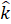Daily Practice Problems
Class 11 Physics
Motion In A PlaneQuestion 1.

Give definitions for vector and scalar quantities with examples.

Question 2.

The position of a particle is given by:

r = 5.0ti+ 2.0t2j+ 6.0kwhere t is in seconds and the coefficients have the proper units for r to be in metres.

1. Find v(t) and a(t) of the particle.
2. Find the magnitude and direction of v(t) at t = 1.0s.

Question 3.

Rain is falling vertically with a speed of 25 m/s. Anita rides a bicycle with a speed of 10 m/s in east to west direction. What is the direction in which she should hold her umbrella?

Question 4.

A man stands on the edge of a cliff 500 m above the ground and throws a stone horizontally with an initial speed of 10 m/s. Neglecting air-resistance, find the time taken by the stone to reach the ground, and the speed with which it hits the ground. (Take g = 9.8 m/s2).

Question 5.

A cricket ball is thrown at a speed of 30 m/s in a direction 300 above the horizontal. Calculate

1. the maximum height,
2. the time taken by the ball to return to the same level,
3. the distance from the thrower to the point where the ball returns to the same level.

Question 6.

What do you understand by a null vector?

Question 7.

Define unit vector.

Question 8.

The position of a particle is given by

r = 4.0ti+ 3.0t2j+ 6.0kwhere t is in seconds and the coefficients have the proper units for r to be in metres.

1. Find the v and a of the particle.
2. What is the magnitude and direction of velocity of the particle at t = 3.0s?

Question 9.

If the velocity of an object changes from v top v’ in time ∆t. What would be the acceleration in this case?

Question 10.

An aircraft is flying at a height of 4000 m above the ground. If the angle subtended at a ground observation point by the aircraft positions 10.0s apart is 300 . What is the speed of the aircraft?

Question 11.

A bullet fired at an angle of 300 with the horizontal hits the ground 6.0 km away. By adjusting its angle of projection, can one hope to hit a target 9.0 km away? Assume that the muzzle speed to be fixed, and neglect air resistance.

Question 12.

What do you understand by projectile motion? Give path, maximum height and range of the projectile.

Question 13.

A bicyclist is riding with a speed of 30 km/h. As he approaches a circular turn on the road of radius 90 m, he applies brakes and reduces his speed at the constant rate of 0.50 m/s every second. What is the magnitude and direction of the net acceleration of the cyclist on the circular turn?

Question 14.

When will you say that an object’s motion is uniform circular motion?

Question 15.

A stone tied to the end of a string 100 cm long is whirled in a horizontal circle with a constant speed. If the stone makes 15 revolutions in 30s, what is the magnitude and direction of acceleration of the stone.

Question 16.

An ant trapped in a circular groove of radius 10cm moves along the groove steadily and completes 5 revolutions in 50s. What is the angular speed and linear speed of the motion?

Question 17.

A cricketer can throw a ball to a maximum horizontal distance of 90m. How much high above the ground can the cricketer throw the same ball?

Question 18.

A particle starts from origin at t=0 with a velocity 6.0im/s and moves in x-y plane under action of a force, which produces a constant acceleration of (4.0i+ 3.0j)m/s2.

1. What is the y- coordinate of the particle at the instant its x-coordinate is 96m?
2. What is the speed of the particle at this time?

Question 19.

A motorboat is racing towards north at 30km/h and the water current in that region is 15km/h in the direction of 300 east of south. Find the resultant velocity of the boat.

Question 20.

Is path length traversed by an object same as the magnitude of displacement?

**********